Jet Set Go! All about Aeroplanes Jet Set Go! All about Aeroplanes

# Important Questions for Class 10 Maths Chapter 8- Introduction to Trigonometry

Important questions of Chapter 8 – Introduction to Trigonometry of Class 10 are given here for students who want to score high marks in their board exams 2022-2023. Students can also download the trigonometry class 10 questions pdf which is available on our website. They can learn and solve the problems offline by downloading trigonometry class 10 questions pdf.  The questions which are provided below are as per the latest CBSE syllabus and are designed according to the NCERT book. The questions are formulated after analyzing the previous year’s questions papers, exam trends and latest sample papers. Solving these questions will help students to get prepared for the final exam. Students can also get important questions for all the chapters of 10th standard Maths. Solve them to get acquainted with the various types of questions to be asked from each chapter of the Maths subject.

Also, get access to Class 10 Maths Chapter 8 Introduction to Trigonometry MCQs here.

In Chapter 8, students will be introduced to the Trigonometry concept, which states the relationship between angles and sides of a triangle. They will come across many trigonometric formulas which will be used to solve numerical problems. The six primary trigonometric ratios are sine, cosine, tangent, secant, cosecant and cotangent. The whole trigonometry concept revolves around these ratios or sometimes also called functions. Students can learn more about trigonometry class 10 questions, which are provided with complete explanations. Trigonometry Class 10 Maths Chapter 8 Important Questions are solved by our expert teachers so that students can understand the problems quickly. Besides, students can also get additional questions on chapter 8 of class 10 maths for practice at the end.

## Class 10 Maths Chapter 8 Important Questions and Answers

Below are the important trigonometry class 10 questions. Students can refer to the below-given class 10 trigonometry questions and they can practice these problems as well.

Question. 1 : In ∆ ABC, right-angled at B, AB = 24 cm, BC = 7 cm.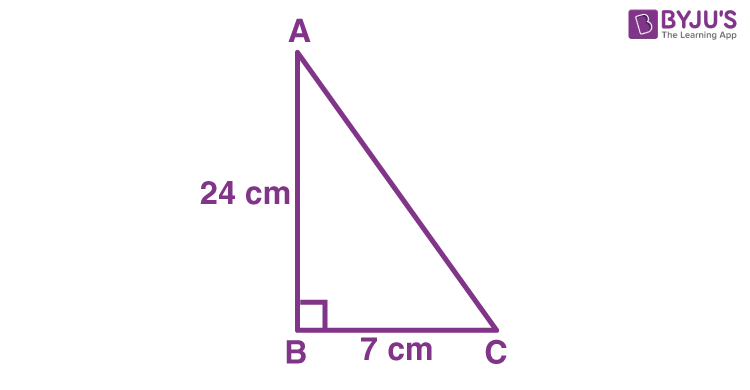Determine:
(i) sin A, cos A
(ii) sin C, cos C

Solution:

In a given triangle ABC, right-angled at B = ∠B = 90°

Given: AB = 24 cm and BC = 7 cm

That means, AC = Hypotenuse

According to the Pythagoras Theorem,

In a right-angled triangle, the squares of the hypotenuse side are equal to the sum of the squares of the other two sides.

By applying the Pythagoras theorem, we get

AC= AB+ BC2

AC2 = (24)+ 72

AC2 = (576 + 49)

AC2 = 625 cm2

Therefore, AC = 25 cm

(i) We need to find Sin A and Cos A.

As we know, sine of the angle is equal to the ratio of the length of the opposite side and hypotenuse side. Therefore,

Sin A = BC/AC = 7/25

Again, the cosine of an angle is equal to the ratio of the adjacent side and hypotenuse side. Therefore,

cos A = AB/AC = 24/25

(ii) We need to find Sin C and Cos C.

Sin C = AB/AC = 24/25

Cos C = BC/AC = 7/25

Question 2: If Sin A = 3/4, Calculate cos A and tan A.

Solution: Let us say, ABC is a right-angled triangle, right-angled at B.

Sin A = 3/4

As we know,

Sin A = Opposite Side/Hypotenuse Side = 3/4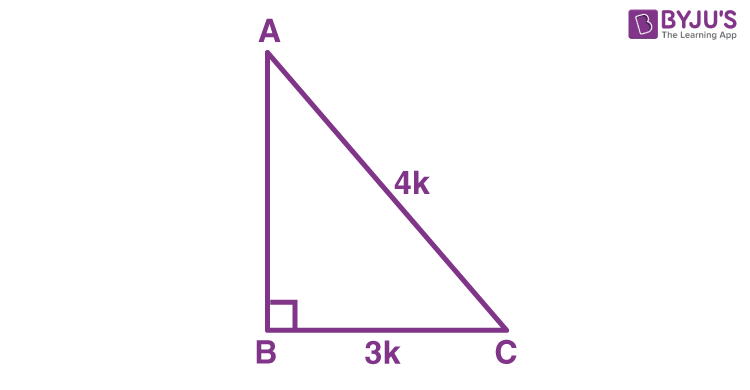Now, let BC be 3k and AC will be 4k.

where k is the positive real number.

As per the Pythagoras theorem, we know;

Hypotenuse2 = Perpendicular2+ Base2

AC2 = AB2 + BC2

Substitute the value of AC and BC in the above expression to get;

(4k)2 = (AB)2 + (3k)2

16k2 – 9k2 = AB2

AB2 = 7k2

Hence, AB = √7 k

Now, as per the question, we need to find the value of cos A and tan A.

cos A = Adjacent Side/Hypotenuse side = AB/AC

cos A = √7 k/4k = √7/4

And,

tan A = Opposite side/Adjacent side = BC/AB

tan A = 3k/√7 k = 3/√7

Question.3: If ∠A and ∠B are acute angles such that cos A = cos B, then show that ∠ A = ∠ B.

Solution:

Suppose a triangle ABC, right-angled at C.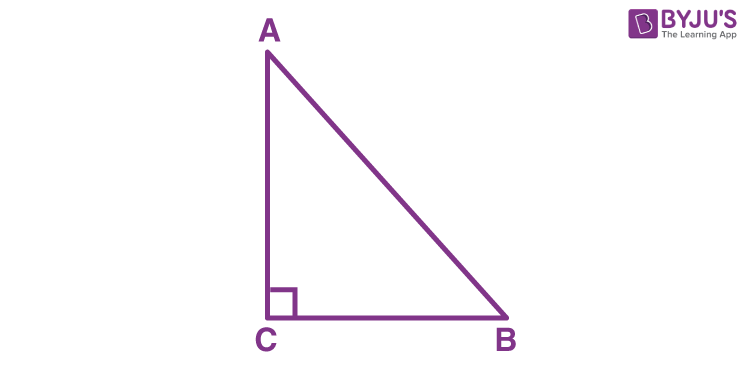Now, we know the trigonometric ratios,

cos A = AC/AB

cos B = BC/AB

Since, it is given,

cos A = cos B

AC/AB = BC/AB

AC = BC

We know that by isosceles triangle theorem, the angles opposite to the equal sides are equal.

Therefore, ∠A = ∠B

Question 4: If 3 cot A = 4, check whether (1 – tan2A)/(1 + tan2A) = cos2 A – sin2 A or not.

Solution:

Let us consider a triangle ABC, right-angled at B.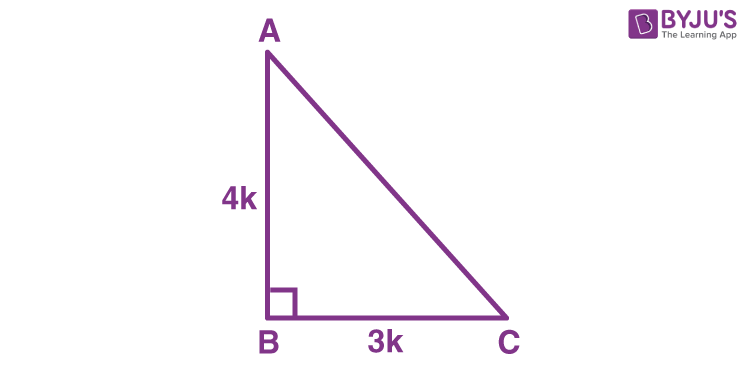Given,

3 cot A = 4

cot A = 4/3

Since, tan A = 1/cot A

tan A = 1/(4/3) = 3/4

BC/AB = 3/4

Let BC = 3k and AB = 4k

By using Pythagoras theorem, we get;

Hypotenuse2 = Perpendicular2 + Base2

AC2 = AB2 + BC2

AC2 = (4k)2 + (3k)2

AC2 = 16k+ 9k2

AC = 25k= 5k

sin A = Opposite side/Hypotenuse

= BC/AC

=3k/5k

=3/5

In the same way,

= AB/AC

= 4k/5k

= 4/5

To check: (1-tan2A)/(1+tan2A) = cos2 A – sin2 A or not

Let us take L.H.S. first;

(1-tan2A)/(1+tan2A) = [1 – (3/4)2]/ [1 + (3/4)2]

= [1 – (9/16)]/[1 + (9/16)] = 7/25

R.H.S. = cos2 A – sin2 A = (4/5)– (3/5)2

= (16/25) – (9/25) = 7/25

Since,

L.H.S. = R.H.S.

Hence, proved.

Question 5: In triangle PQR, right-angled at Q, PR + QR = 25 cm and PQ = 5 cm. Determine the values of sin P, cos P and tan P.

Solution: Given,

In triangle PQR,

PQ = 5 cm

PR + QR = 25 cm

Let us say, QR = x

Then, PR = 25 – QR = 25 – x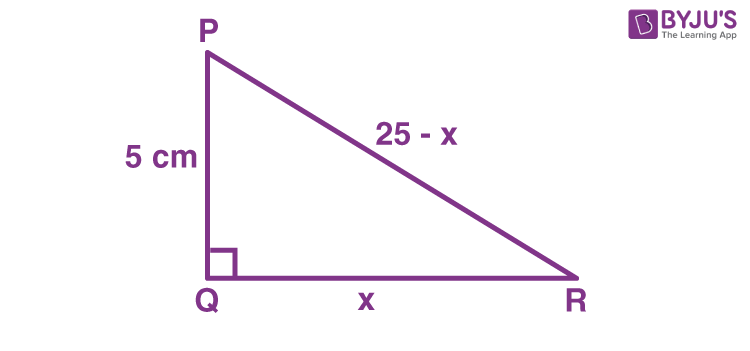Using Pythagoras theorem:

PR2 = PQ2 + QR2

Now, substituting the value of PR, PQ and QR, we get;

(25 – x)2 = (5)2 + (x)2

25+ x– 50x = 25 + x2

625 – 50x = 25

50x = 600

x = 12

So, QR = 12 cm

PR = 25 – QR = 25 – 12 = 13 cm

Therefore,

sin P = QR/PR = 12/13

cos P = PQ/PR = 5/13

tan P = QR/PQ = 12/5

Question 6: Evaluate 2 tan2 45° + cos2 30° – sin2 60°.

Solution: Since we know,

tan 45° = 1

cos 30° = 3/2

sin 60° = 3/2

Therefore, putting these values in the given equation:

2(1)2 + (3/2)2 – (3/2)2

= 2 + 0

= 2

Question 7: If tan (A + B) =√3 and tan (A – B) =1/√3, 0° < A + B ≤ 90°; A > B, find A and B.

Solution: Given,

tan (A + B) = √3

As we know, tan 60° = √3

Thus, we can write;

⇒ tan (A + B) = tan 60°

⇒(A + B) = 60° …… (i)

Now again given;

tan (A – B) = 1/√3

Since, tan 30° = 1/√3

Thus, we can write;

⇒ tan (A – B) = tan 30°

⇒(A – B) = 30° ….. (ii)

Adding the equation (i) and (ii), we get;

A + B + A – B = 60° + 30°

2A = 90°

A= 45°

Now, put the value of A in eq. (i) to find the value of B;

45° + B = 60°

B = 60° – 45°

B = 15°

Therefore A = 45° and B = 15°

Question 8: Show that :

(i) tan 48° tan 23° tan 42° tan 67° = 1

(ii) cos 38° cos 52° – sin 38° sin 52° = 0

Solution:

(i) tan 48° tan 23° tan 42° tan 67°

We can also write the above given tan functions in terms of cot functions, such as;

tan 48° = tan (90° – 42°) = cot 42°

tan 23° = tan (90° – 67°) = cot 67°

Hence, substituting these values, we get

= cot 42° cot 67° tan 42° tan 67°

= (cot 42° tan 42°) (cot 67° tan 67°)

= 1 × 1 [since cot A.tan A = 1]

= 1

(ii) cos 38° cos 52° – sin 38° sin 52°

We can also write the given cos functions in terms of sin functions.

cos 38° = cos (90° – 52°) = sin 52°

cos 52°= cos (90° – 38°) = sin 38°

Hence, putting these values in the given equation, we get;

sin 52° sin 38° – sin 38° sin 52° = 0

Question 9: If tan 2A = cot (A – 18°), where 2A is an acute angle, find the value of A.

Solution: Given,

tan 2A = cot (A – 18°)

As we know by trigonometric identities,

tan 2A = cot (90° – 2A)

Substituting the above equation in the given equation, we get;

⇒ cot (90° – 2A) = cot (A – 18°)

Therefore,

⇒ 90° – 2A = A – 18°

⇒ 108° = 3A

A = 108° / 3

Hence, the value of A = 36°

Question 10:  If A, B and C are interior angles of a triangle ABC, then show that sin [(B + C)/2] = cos A/2.

Solution:

As we know, for any given triangle, the sum of all its interior angles is equals to 180°.

Thus,

A + B + C = 180° ….(1)

Now we can write the above equation as;

⇒ B + C = 180° – A

Dividing by 2 on both the sides;

⇒ (B + C)/2 = (180° – A)/2

⇒ (B + C)/2 = 90° – A/2

Now, put sin function on both sides.

⇒ sin (B + C)/2 = sin (90° – A/2)

Since,

sin (90° – A/2) = cos A/2

Therefore,

sin (B + C)/2 = cos A/2

Question 11: Prove the identities:

(i) √[1 + sinA/1 – sinA] = sec A + tan A

(ii) (1 + tan2A/1 + cot2A) = (1 – tan A/1 – cot A)2 = tan2A
Solution:
(i) Given:√[1 + sinA/1 – sinA] = sec A + tan A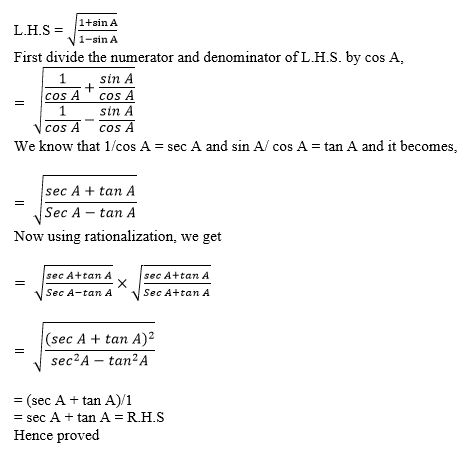(ii) Given: (1 + tan2A/1 + cot2A) = (1 – tan A/1 – cot A)2 = tan2A

LHS:
= (1+tan²A) / (1+cot²A)
Using the trigonometric identities we know that 1+tan²A = sec²A and 1+cot²A= cosec²A
= sec²A/ cosec²A
On taking the reciprocals we get
= sin²A/cos²A
= tan²A
RHS:
=(1-tanA)²/(1-cotA)²
Substituting the reciprocal value of tan A and cot A we get,
= (1-sinA/cosA)²/(1-cosA/sinA)²
= [(cosA-sinA)/cosA]²/ [(sinA-cos)/sinA)²] = [(cosA-sinA)²×sin²A] /[cos²A. /(sinA-cosA)²] =  sin²A/cos²A
= tan2A
The values of LHS and RHS are the same.
Hence proved.

Question 12: If sin θ + cos θ = √3, then prove that tan θ + cot θ = 1.

Solution:

Given,

sin θ + cos θ = √3

Squaring on both sides,

(sin θ + cos θ)2 = (√3)2

sin2θ + cos2θ + 2 sin θ cos θ = 3

Using the identity sin2A + cos2A = 1,

1 + 2 sin θ cos θ = 3

2 sin θ cos θ = 3 – 1

2 sin θ cos θ = 2

sin θ cos θ = 1

sin θ cos θ = sin2θ + cos2θ

⇒ (sin2θ + cos2θ)/(sin θ cos θ) = 1

⇒ [sin2θ/(sin θ cos θ)] + [cos2θ/(sin θ cos θ)] = 1

⇒ (sin θ/cos θ) + (cos θ/sin θ) = 1

⇒ tan θ + cot θ = 1

Hence proved.

Question 13: Express cot 85° + cos 75° in terms of trigonometric ratios of angles between 0° and 45°.

Solution:

cot 85° + cos 75°

= cot (90° – 5°) + cos (90° – 15°)

We know that cos(90° – A) = sin A and cot(90° – A) = tan A

= tan 5° + sin 15°

Question 14: What is the value of (cos267° – sin223°)?

Solution:

(cos267° – sin223°) = cos2(90° – 23°) – sin223°

We know that cos(90° – A) = sin A

= sin223° – sin223°

= 0

Therefore, (cos267° – sin223°) = 1.

Question 15: Prove that (sin A – 2 sin3A)/(2 cos3A – cos A) = tan A.

Solution:

LHS = (sin A – 2 sin3A)/(2 cos3A – cos A)

= [sin A(1 – 2 sin2A)]/ [cos A(2 cos2A – 1]

Using the identity sin2θ + cos2θ = 1,

= [sin A(sin2A + cos2A – 2 sin2A)]/ [cos A(2 cos2A – sin2A – cos2A]

= [sin A(cos2A – sin2A)]/ [cos A(cos2A – sin2A)]

= sin A/cos A

= tan A

= RHS

Hence proved.

Also, check: Trigonometry Class 10 Questions PDF.

## Video Lesson on Trigonometry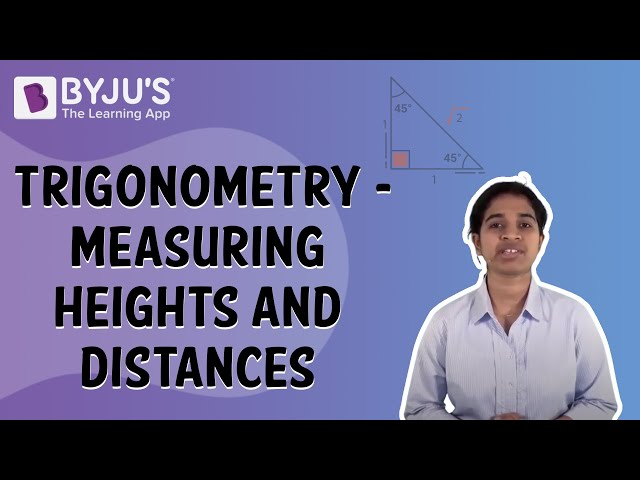### Introduction to Trigonometry Class 10 Questions for Practice

Solve the following class 10 Maths trigonometry problems:

1. If sec θ + tan θ = 7, the compute the value of sec θ – tan θ
2. If tan θ + cot θ = 5, then find the value of tan2θ + cot2θ.
3. What will happen if the value of the cosine function increases from 0° to 90°?
4. Given that cosec θ = 4/3. Find all other values of trigonometric ratios.
5. Find the value of sin 45 °- Cos 45°.
6. If 4 tan θ = 3, evaluate (4 sin θ – cos θ + 1)/(4 sin θ + cos θ + 1).
7. Prove that [tan θ/(1 – cot θ)] + [cot θ/(1 – tan θ)] = 1 + sec θ cosec θ
8. Prove that: sin θ/(cot θ + cosec θ) = 2 + [sin θ/ (cot θ – cosec θ]
9. In a right triangle ABC, right-angled at B, if tan A = 1, then verify that 2 sin A cos A = 1.
10. If ∠B and ∠Q are acute angles such that sin B = sin Q, then prove that ∠B = ∠Q.

We hope students must have found this information on Important Questions Class 10 Maths Chapter 8 Introduction to Trigonometry helpful for their board exam preparation. Stay tuned with BYJU’S – The Learning App and download the app to get all the chapter-wise important questions for class 10 Maths and also get the latest updates on the syllabus.

Nice work by byjus ..

3. It is very good work from byjus and this questions are very helpful for us

4. Good short and easy but important one’s

thank u soo much byju

6. Rachana

That’s very helpful to me during my exams. Thanks to BYJU’S App.

Thank you byjus. I was searching for trigonometry related this type questions only.

9. Cetna DJ

Thank you Byju’s the learning app,this is going to be much helpful for us
Please upload more questions similar to this with a little bit more complexity.
By the way, I am one of those Byju’s offline users, but yet searching in google
for more questions
Please upload the most important EXERCISE QUESTIONS and EXAMPLE QUESTIONS from textbook as well

10. Maanya Bhatia

Thank you Byju’s this helped me a lot in my internal assessments.

11. Thank you, BYJU’S ! It helped me a lot for my PRE-BOARD.